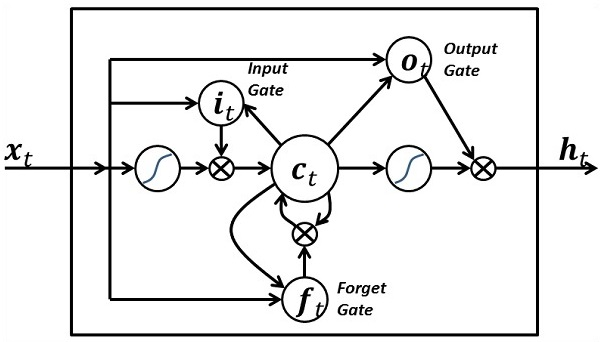# Recommendations for Neural Network Training

In this chapter, we will understand the various aspects of neural network training which can be implemented using TensorFlow framework.

Following are the ten recommendations, which can be evaluated −

## Back Propagation

Back propagation is a simple method to compute partial derivatives, which includes the basic form of composition best suitable for neural nets.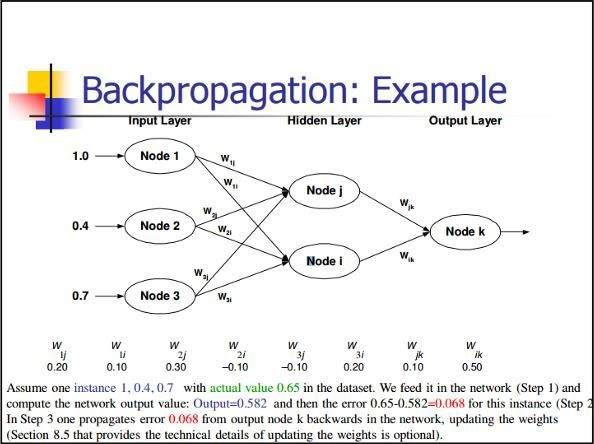In stochastic gradient descent, a batch is the total number of examples, which a user uses to calculate the gradient in a single iteration. So far, it is assumed that the batch has been the entire data set. The best illustration is working at Google scale; data sets often contain billions or even hundreds of billions of examples.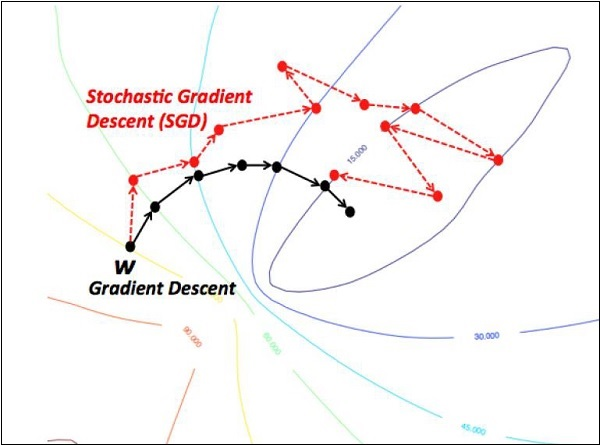## Learning Rate Decay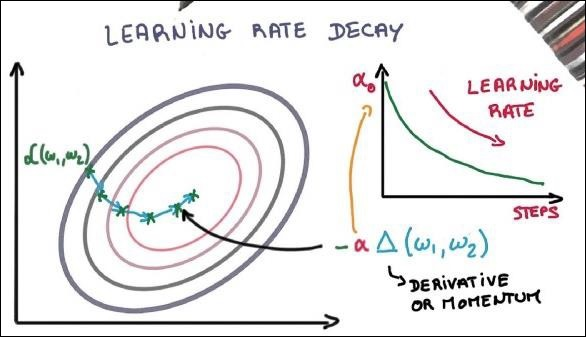Adapting the learning rate is one of the most important features of gradient descent optimization. This is crucial to TensorFlow implementation.

## Dropout

Deep neural nets with a large number of parameters form powerful machine learning systems. However, over fitting is a serious problem in such networks.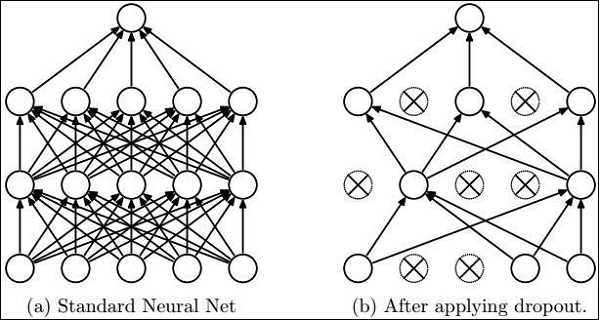## Max Pooling

Max pooling is a sample-based discretization process. The object is to down-sample an input representation, which reduces the dimensionality with the required assumptions.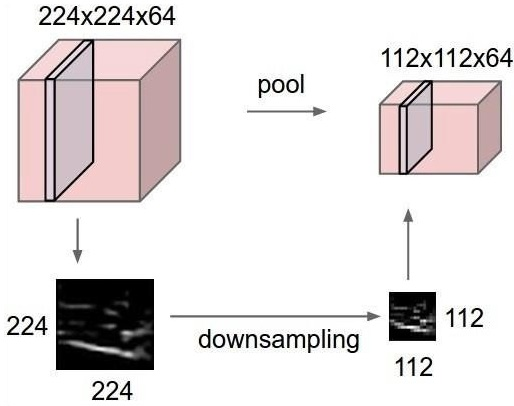## Long Short Term Memory (LSTM)

LSTM controls the decision on what inputs should be taken within the specified neuron. It includes the control on deciding what should be computed and what output should be generated.